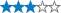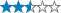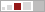##### This cache is temporarily unavailable.

A2G: We have no idea is this is findable or not. The puzzle is still in tact, but the cache may not be. We will find someone to repair or archive soon. Hate to see this awesome yurtles puzzle die, but it may be time. :(

More
<

A cache by yurtles adopted by A2G Message this owner
Hidden : 02/04/2013
Difficulty:Terrain:Size:(regular)

#### Watch

How Geocaching WorksPlease note Use of geocaching.com services is subject to the terms and conditions in our disclaimer.

### Geocache Description:

The given coordinates are for parking. If you park on Bliss Road, the terrain rating for the cache would go up dramatically and, worse yet, the Corps of Engineers would rescind our permit for the cache. Please don't park on Bliss Road!
===833=242=233=121=225=425=1105=162=2103=373=553=742=176====
The cache is nowhere near the given coordinates. You'll have to find the puzzle and solve it in order to find out where the ammo can is hidden.
===389=5102=431=863=6110=141=534=713=852=4611=753=363=264===
Ghosts are all around us. On the south shore of Cowanesque Lake you can see the ghost of old route 49. On the north shore, you can see the ghost of a railroad. There's even a sign to tell you you're close.
===7124=6105=5121=654=872=541=483=735=5141=154=3102=621======215=416=7113=131=441=252=281=663=845=783=344=8117======677=354=1113=575=295=315=171=895=6116=561=825=389=187===Good luck and happy caching.

CONGRATULATIONS! FTF to TEAM SparkleA2jeskyCEHR+A. Very well done!

===yvarjbeqpunenpgre===

Decryption Key

A|B|C|D|E|F|G|H|I|J|K|L|M
-------------------------
N|O|P|Q|R|S|T|U|V|W|X|Y|Z

(letter above equals below, and vice versa)

# Reviewer notes

Use this space to describe your geocache location, container, and how it's hidden to your reviewer. If you've made changes, tell the reviewer what changes you made. The more they know, the easier it is for them to publish your geocache. This note will not be visible to the public when your geocache is published.